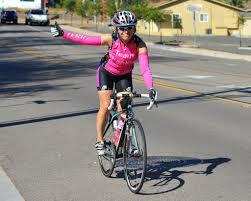# Cyclo trip

Eighth-graders took a full-day trip on a cycling course. They took 1/7 of the route to the first break and added another 3/7 of the route to lunch. They had 18 km left to the finish. How many kilometers did the trip route measure?

x =  42 km

### Step-by-step explanation:

1/7 x + 3/7x + 18 =x

1/7·x + 3/7·x + 18 =x

3x = 126

x = 126/3 = 42

x = 42

Our simple equation calculator calculates it.Did you find an error or inaccuracy? Feel free to write us. Thank you!

Tips for related online calculators
Need help calculating sum, simplifying, or multiplying fractions? Try our fraction calculator.
Do you have a linear equation or system of equations and looking for its solution? Or do you have a quadratic equation?